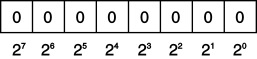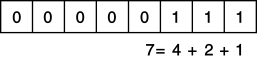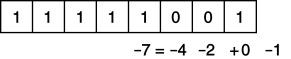Bitwise Operators (<<, >>, |, &, ^, ~)

An additional set of operators that is common to virtually all programming languages is the set of operators for manipulating values in their binary formats: the bit operators.Beginner Topic
Bits and Bytes

All values within a computer are represented in a binary format of 1s and 0s, called binary digits (bits). Bits are grouped together in sets of eight, called bytes. In a byte, each successive bit corresponds to a value of 2 raised to a power, starting from 20 on the right and moving to 27 on the left, as shown in Figure 4.1.Figure 4.1: Corresponding placeholder values

In many scenarios, particularly when dealing with low-level or system services, information is retrieved as binary data. To manipulate these devices and services, you need to perform manipulations of binary data.

In Figure 4.2, each box corresponds to a value of 2 raised to the power shown. The value of the byte (8-bit number) is the sum of the powers of 2 of all of the eight bits that are set to 1.Figure 4.2: Calculating the value of an unsigned byte

The binary translation just described is significantly different for signed numbers. Signed numbers (long, short, int) are represented using a two’s complement notation. This practice ensures that addition continues to work when adding a negative number to a positive number, as though both were positive operands. With this notation, negative numbers behave differently from positive numbers. Negative numbers are identified by a 1 in the leftmost location. If the leftmost location contains a 1, you add the locations with 0s rather than the locations with 1s. Each location corresponds to the negative power of 2 value. Furthermore, from the result, it is also necessary to subtract 1. This is demonstrated in Figure 4.3.Figure 4.3: Calculating the value of a signed byte

Therefore, 1111 1111 1111 1111 corresponds to –1, and 1111 1111 1111 1001 holds the value –7. The binary representation 1000 0000 0000 0000 corresponds to the lowest negative value that a 16-bit integer can hold.

Shift Operators (<<, >>, <<=, >>=)

Sometimes you want to shift the binary value of a number to the right or left. In executing a left shift, all bits in a number’s binary representation are shifted to the left by the number of locations specified by the operand on the right of the shift operator. Zeroes then backfill the locations on the right side of the binary number. A right-shift operator does almost the same thing in the opposite direction. However, if the number is a negative value of a signed type, the values used to backfill the left side of the binary number are 1s, rather than 0s. The shift operators are >> and <<, known as the right-shift and left-shift operators, respectively. In addition, C# includes combination shift and assignment operators, <<= and >>=.

Consider the following example. Suppose you had the int value -7, which would have a binary representation of 1111 1111 1111 1111 1111 1111 1111 1001. In Listing 4.40 (with Output 4.17), you right-shift the binary representation of the number –7 by two locations.

Listing 4.40: Using the Right-Shift Operator
int x;
x = (-7 >> 2); // 11111111111111111111111111111001 becomes
// 11111111111111111111111111111110
// Write out "x is -2."
Console.WriteLine(\$"x = {x}.");
Output 4.17
x = -2.

Because of the right shift, the value of the bit in the rightmost location has “dropped off” the edge, and the negative bit indicator on the left shifts by two locations to be replaced with 1s. The result is -2.

Although legend has it that x << 2 is faster than x * 4, you should not use bit-shift operators for multiplication or division. This difference might have held true for certain C compilers in the 1970s, but modern compilers and modern microprocessors are perfectly capable of optimizing arithmetic. Using shifting for multiplication or division is confusing and frequently leads to errors when code maintainers forget that the shift operators are lower precedence than the arithmetic operators.

Bitwise Operators (&, |, ^)

In some instances, you might need to perform logical operations, such as AND, OR, and XOR, on a bit-by-bit basis for two operands. You do this via the &, |, and ^ operators, respectively.Beginner Topic
Logical Operators Explained

If you have two numbers, as shown in Figure 4.4, the bitwise operations will compare the values of the locations beginning at the leftmost significant value and continuing right until the end. The value of “1” in a location is treated as “true,” and the value of “0” in a location is treated as “false.”Figure 4.4: The numbers 12 and 7 represented in binary

The bitwise AND of the two values in Figure 4.4 would entail the bit-by-bit comparison of bits in the first operand (12) with the bits in the second operand (7), resulting in the binary value 000000100, which is 4. Alternatively, a bitwise OR of the two values would produce 00001111, the binary equivalent of 15. The XOR result would be 00001011, or decimal 11.

Listing 4.41 demonstrates the use of these bitwise operators. The results of Listing 4.41 appear in Output 4.18.

Listing 4.41: Using Bitwise Operators
byte andor, xor;
and = 12 & 7;   // and = 4
or = 12 | 7;    // or = 15
xor = 12 ^ 7;   // xor = 11
Console.WriteLine( \$"""
and = { and }
or = { or }
xor = { xor }
""");
Output 4.18
and = 4
or = 15
xor = 11

In Listing 4.41, the value 7 is the mask; it is used to expose or eliminate specific bits within the first operand using the specified operator expression. Note that, unlike the AND (&&) operator, the & operator always evaluates both sides even if the left portion is false. Similarly, the | version of the OR operator is not “short-circuiting”: It always evaluates both operands, even if the left operand is true. The bit versions of the AND and OR operators, therefore, are not short-circuiting.

To convert a number to its binary representation, you need to iterate across each bit in a number. Listing 4.42 is an example of a program that converts an integer to a string of its binary representation. The results of Listing 4.42 appear in Output 4.19.

Listing 4.42: Getting a String Representation of a Binary Display
public class BinaryConverter
{
public static void Main()
{
const int size = 64;
ulong value;
char bit;

Console.Write("Enter an integer: ");
// Use long.Parse() to support negative numbers
// Assumes unchecked assignment to ulong
// If ReadLine returns null, use "42" as default input

// Set initial mask to 100....
ulong mask = 1UL << size - 1;
for(int count = 0; count < size; count++)
{
bit = ((mask & value) != 0) ? '1' : '0';
Console.Write(bit);
// Shift mask one location over to the right
}
Console.WriteLine();
}
}
Output 4.19
Enter an integer: 42
0000000000000000000000000000000000000000000000000000000000101010

Within each iteration of the for loop in Listing 4.42 (as discussed later in this chapter), we use the right-shift assignment operator to create a mask corresponding to each bit position in value. By using the & bit operator to mask a particular bit, we can determine whether the bit is set. If the mask test produces a nonzero result, we write 1 to the console; otherwise, we write 0. In this way, we create output describing the binary value of an unsigned long.

Note also that the parentheses in (mask & value) != 0 are necessary because inequality is higher precedence than the AND operator. Without the explicit parentheses, this expression would be equivalent to mask & (value != 0), which does not make any sense; the left side of the & is a ulong and the right side is a bool.

This example is provided for learning purposes only. There is actually a built-in CLR method, System.Convert.ToString(value, 2), that does such a conversion. In fact, the second argument specifies the base (e.g., 2 for binary, 10 for decimal, or 16 for hexadecimal), allowing for more than just conversion to binary.

Bitwise Compound Assignment Operators (&=, |=, ^=)

Not surprisingly, you can combine these bitwise operators with assignment operators as follows: &=, |=, and ^=. As a result, you could take a variable, OR it with a number, and assign the result back to the original variable, which Listing 4.43 with Output 4.20 demonstrates.

Listing 4.43: Using Logical Assignment Operators
byte and = 12, or = 12, xor = 12;
and &= 7;   // and = 4
or |= 7;   // or = 15
xor ^= 7;   // xor = 11
Console.WriteLine( \$"""
and = {and}
or = {or}
xor = {xor}
""");
Output 4.20
and = 4
or = 15
xor = 11

Combining a bitmap with a mask using something like fields &= mask clears the bits in fields that are not set in the mask. The opposite, fields &= ~mask, clears the bits in fields that are set in mask.

Bitwise Complement Operator (~)

The bitwise complement operator takes the complement of each bit in the operand, where the operand can be an int, uint, long, or ulong. The expression ~1, therefore, returns the value with binary notation 1111 1111 1111 1111 1111 1111 1111 1110, and ~(1<<31) returns the number with binary notation 0111 1111 1111 1111 1111 1111 1111 1111.

{{ snackbarMessage }}
• {{item.title}}
• {{item.title}}